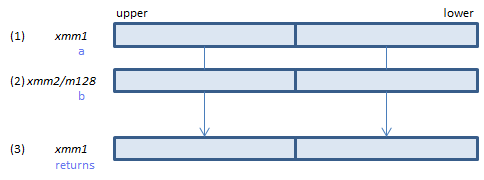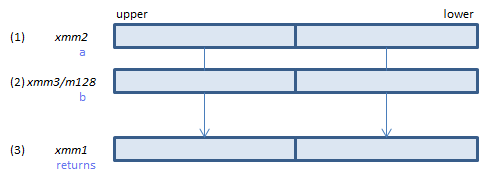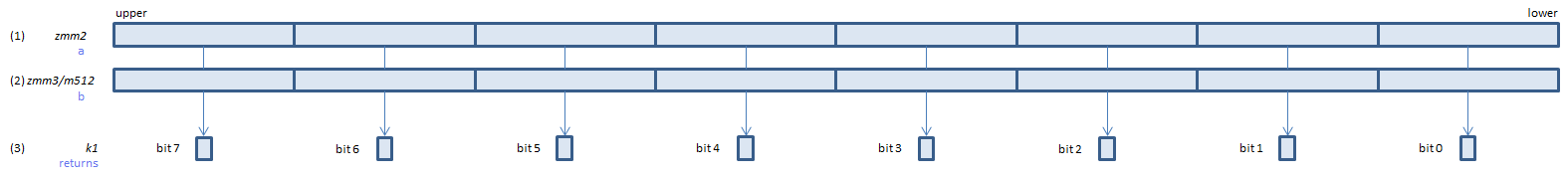﻿ cmpeqpd

## CMPEQPD - CoMPare EQual Packed Double

CMPEQPD xmm1, xmm2/m128    (S2
__m128d _mm_cmpeq_pd(__m128d a, __m128d b)For each double, if (1) == (2) set 1, else set 0, to all bits of the corresponding double of (3).
VCMPEQPD xmm1, xmm2, xmm3/m128    (V1
__m128d _mm_cmpeq_pd(__m128d a, __m128d b)For each double, if (1) == (2) set 1, else set 0, to all bits of the corresponding double of (3).
VCMPEQPD ymm1, ymm2, ymm3/m256    (V1
__m256d _mm256_cmp_pd(__m256d a, __m256d b, _CMP_EQ_OQ)For each double, if (1) == (2) set 1, else set 0, to all bits of the corresponding double of (3).
VCMPEQPD k1{k2}, xmm2, xmm3/m128/m64bcst    (V5+VLFor each double, if (1) == (2) set 1, else set 0, to the corresponding bit of (3).
If k2 bit is 0, the comparison is not done and the corresponding bit of (3) is set to zero. Upper bits of (3) are zero cleared.
VCMPEQPD k1{k2}, ymm2, ymm3/m256/m64bcst    (V5+VLFor each double, if (1) == (2) set 1, else set 0, to the corresponding bit of (3).
If k2 bit is 0, the comparison is not done and the corresponding bit of (3) is set to zero. Upper bits of (3) are zero cleared.
VCMPEQPD k1{k2}, zmm2, zmm3/m512/m64bcst{sae}    (V5